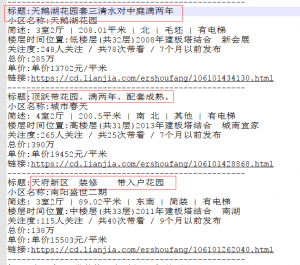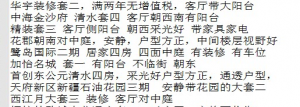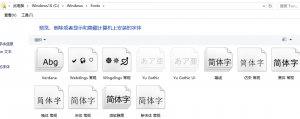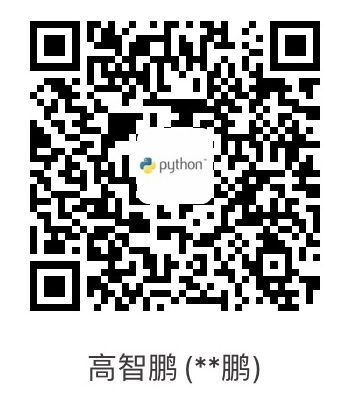# 对成都二手房数据进行可视化分析后，我发现……

### 对成都二手房数据进行可视化分析后，我发现……```with open('chengdu-lianjia.txt','r',encoding='utf-8')as f,open('biaoti.txt','w',encoding='utf-8')as e:
if '标题' in i:
e.write(i[3:])``````import jieba
from PIL import Image
from collections import Counter
from wordcloud import WordCloud

def get_words(text):
word_dict = {}
seg_list = jieba.cut(text)
c = Counter()
for x in seg_list:
if len(x) &gt; 1 and x != '\r\n':
c[x] += 1
print('正在进行词频分析.....')
for (k, v) in c.most_common(100):
word_dict[k] = v
return word_dict

with open('biaoti.txt', 'r',encoding='utf-8') as f:
frequencies=get_words(text)
wc = WordCloud(font_path=r'C:\Windows\Fonts\simsun.ttc',width=800,height=400,
max_words=200,background_color='white')
wc.generate_from_frequencies(frequencies)
wc.to_file(r'wordcloud.png')
Image.open(r'wordcloud.png','r').show()``````from pyecharts import Bar
from collections import Counter

def get_words(text):
word_list = []
word_dict = {}
seg_list = text
c = Counter()
for x in seg_list:
if len(x) &gt; 1 :
c[x] += 1
print('正在进行词频分析.....')
for (k, v) in c.most_common(100):
word_dict[k] = v
communities = (k,v)
word_list.append(communities)
print(word_dict)
return word_dict,word_list

with open('community.txt','r',encoding='utf-8')as f:
words_dict,words_list = get_words(text)

community_list = words_list[:20]
community,num =[],[]
for i in range(len(community_list)):
community.append(community_list[i][:-2])
num.append(community_list[i])

bar = Bar('二手房小区数量图','成都')
bar.render()```

```from pyecharts import Pie

h,num = {},0
with open('chengdu-lianjia.txt','r',encoding='utf-8')as f:
if '简述' in i:
huxing = i.split('|')[4:-1]
if huxing not in h:
h[huxing] = 1
else:
h[huxing] +=1
for i in list(h.keys()):
if h.get(i) &lt; 50:
num +=h.get(i)
del h[i]
h['其他'] = num
key,value = list(h.keys()),list(h.values())
pie = Pie('户型比例')
pie.render('pie.html')```

# 还是洗洗睡吧！微信赞赏支付宝赞赏